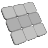Back to Adaptive Vision Library website

You are here: Start » Function Reference » Principal Component Analysis

# Principal Component Analysis

Select a function from the list below.

IconNameDescriptionApplyPCATransformApplies previously obtained Principal Component Analysis (PCA) transformation coefficients to new data.CreatePCATransformPerforms the Principal Component Analysis (PCA) on provided data, creates the feature vector and normalization coefficients (mean and standard deviation of variables).MatrixDeterminantFind the determinant of a square matrix.MatrixPseudoEigenvectorsFind the pseudo-eigenvalues and pseudo-eigenvectors of a symmetrical square matrix.
NormalizeMatrixDataTreats Matrix as a data frame, where examples are in rows while columns represent features, and normalizes the data by subtracting mean from each column and dividing it by its standard deviation.ReversePCATransformReverses Principal Component Analysis (PCA) process. Can be used to transform data back to original feature space.